# How to calculate hours worked in Google Sheets

In this tutorial, we will learn how to calculate hours worked in Google Sheets. In Google Sheets, you can use various built-in functions and formulas to calculate the total hours worked by employees and track employee work hours and productivity over time. This is typically done by subtracting the start time of an employee’s shift from the end time, and then calculating the difference as hours and minutes worked.

For instance, we have a data set representing starting and ending times of an employee for a week. We will calculate hours worked for each day and then for the whole week.Determining the total amount of time an employee has worked during a specific period is referred to as calculating hours worked in Google Sheets. This process is crucial as it can simplify and streamline the tracking of employee work hours, ensure accurate payment for hours worked, and identify areas where employee productivity can be improved.

### Step 1 – Select a Blank Cell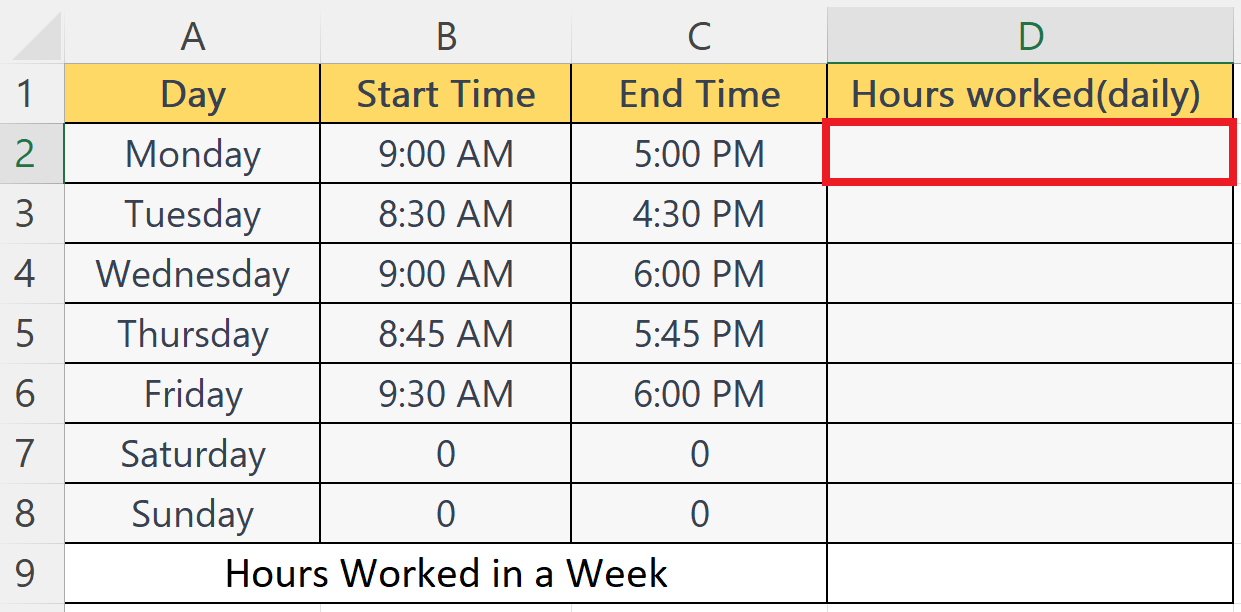– Select a blank cell where you want to calculate hours worked (daily).

### Step 2 – Place an Equals Sign– Place an Equals sign in the blank cell.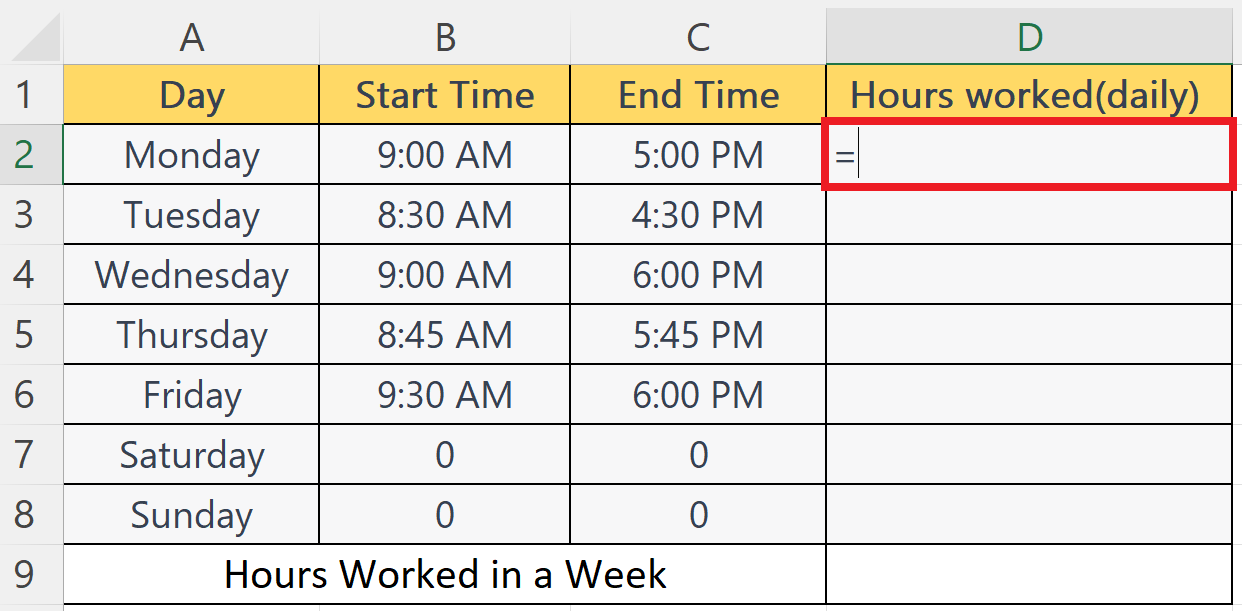### Step 3 – Subtract the Start Time from the End Time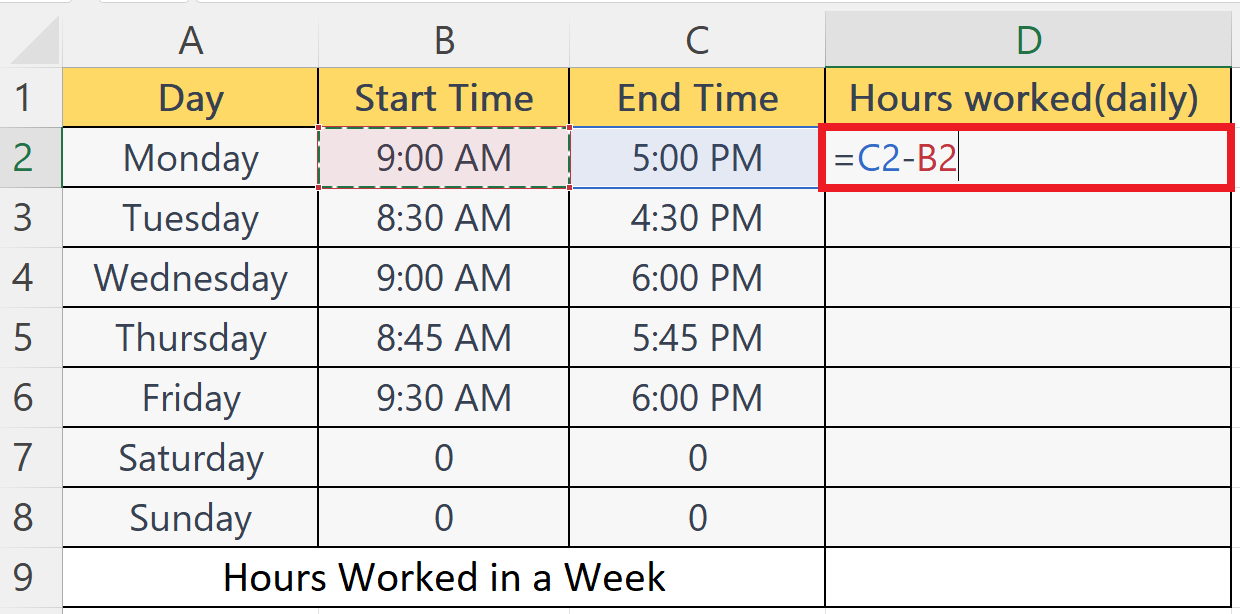– Subtract the start time from the end time i.e. C2-B2.
– Where C2 and B2 are the cells containing the end time and start time respectively.

### Step 4 – Multiple by 24– Enclose the formulae in parentheses.
– Multiply the whole formulae by 24.

### Step 5 – Press the Enter Key– Press the Enter key to calculate the hours worked.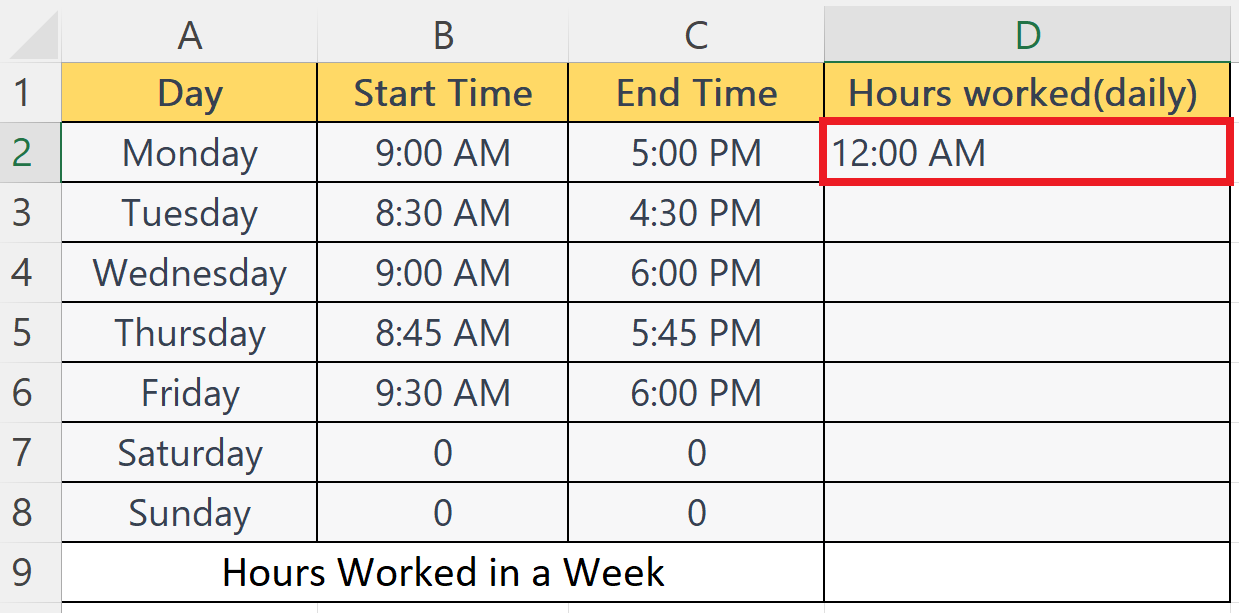– Press the Enter key to calculate the hours worked.

### Step 6 – Change the Format of the Cell to Number Format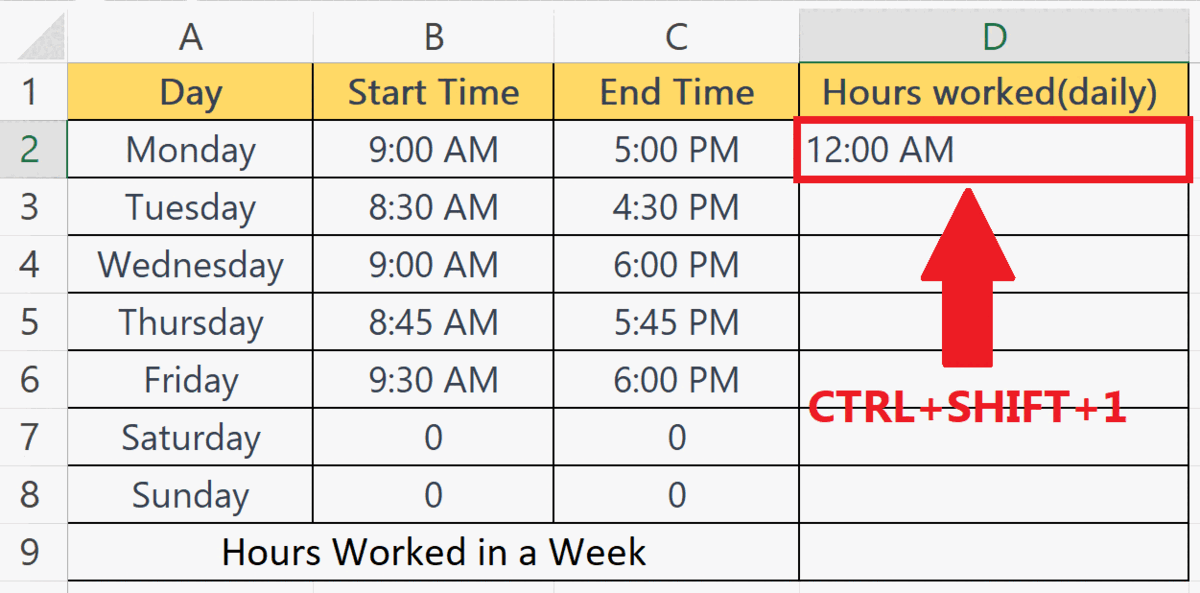– Change the format of the cell to Number format.
– This can be done using the Format Cell option in the context menu or simply by pressing the CTRL+SHIFT+1 shortcut keys.

### Step 7 – Use Autofill to Calculate the Hours Worked for Each Day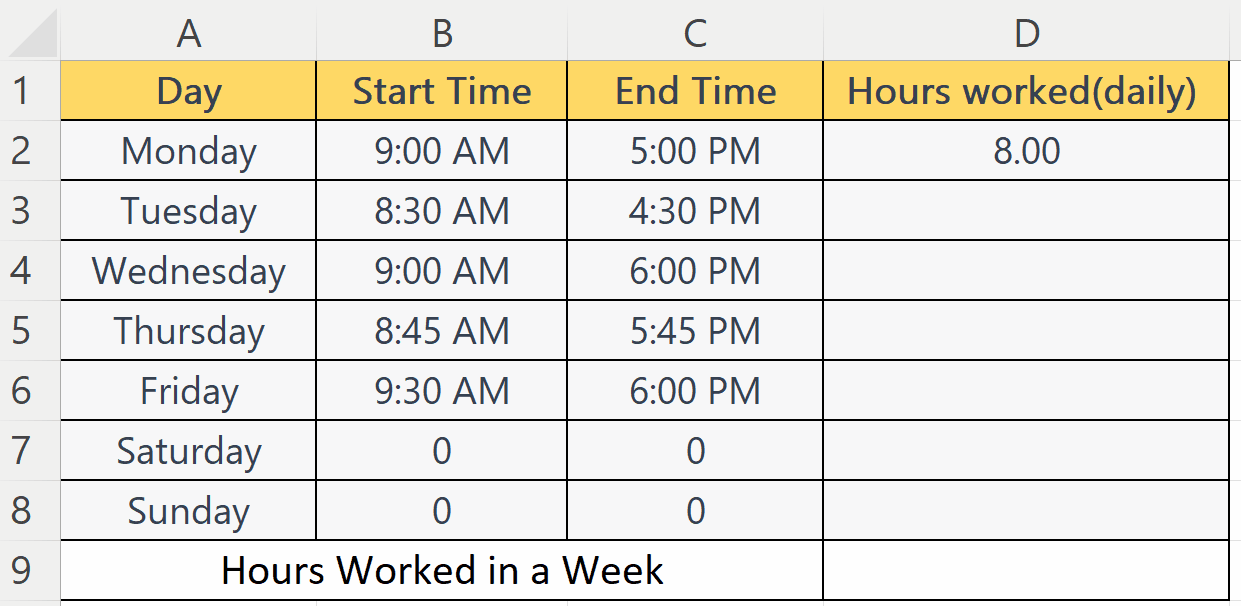– Use Autofill to calculate hours worked for each day.

### Step 8 – Use the SUM Function to Calculate the Hours Worked in a Week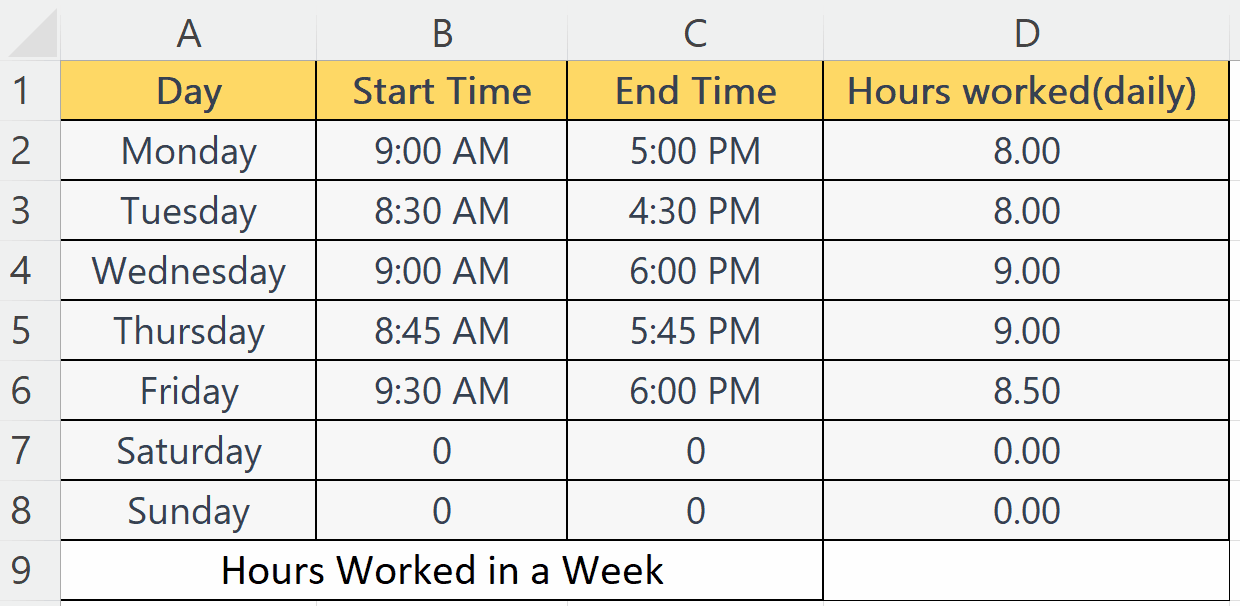– Select a blank cell where you want to calculate weekly hours worked.
– Enter the SUM function.
– The syntax will become:
SUM(D2:D8)
– Where the D2:D8 is the range containing the hours worked for each day in the week.

### Step 9 – Press the Enter Key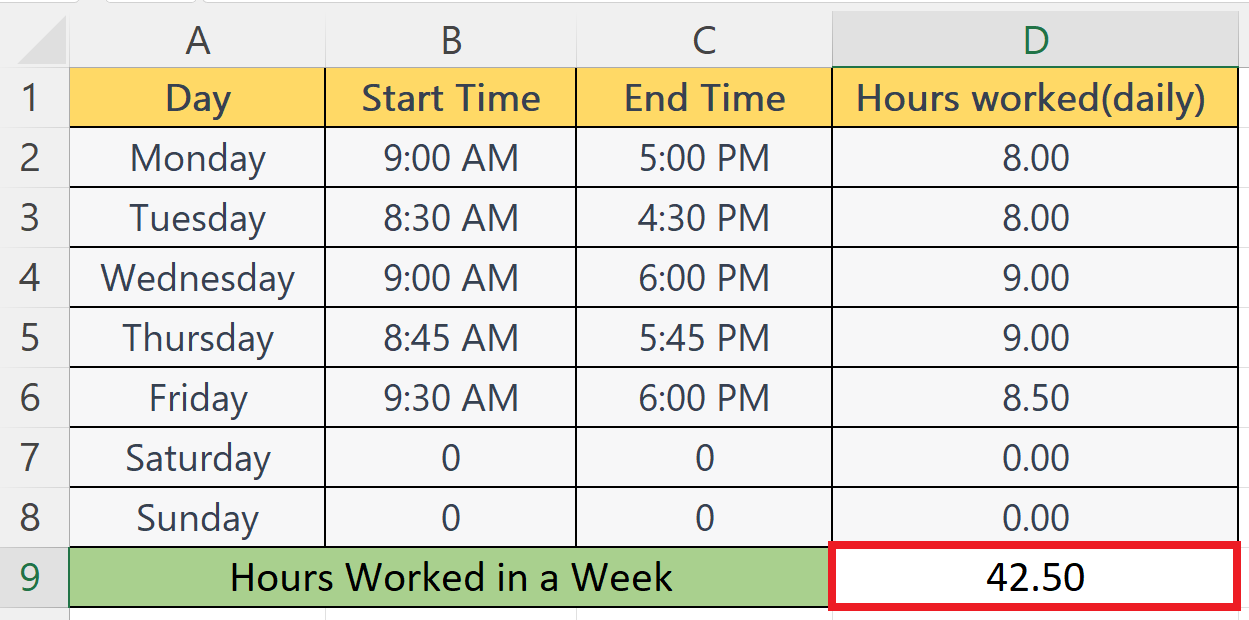– Press the Enter key to calculate hours worked in the week.